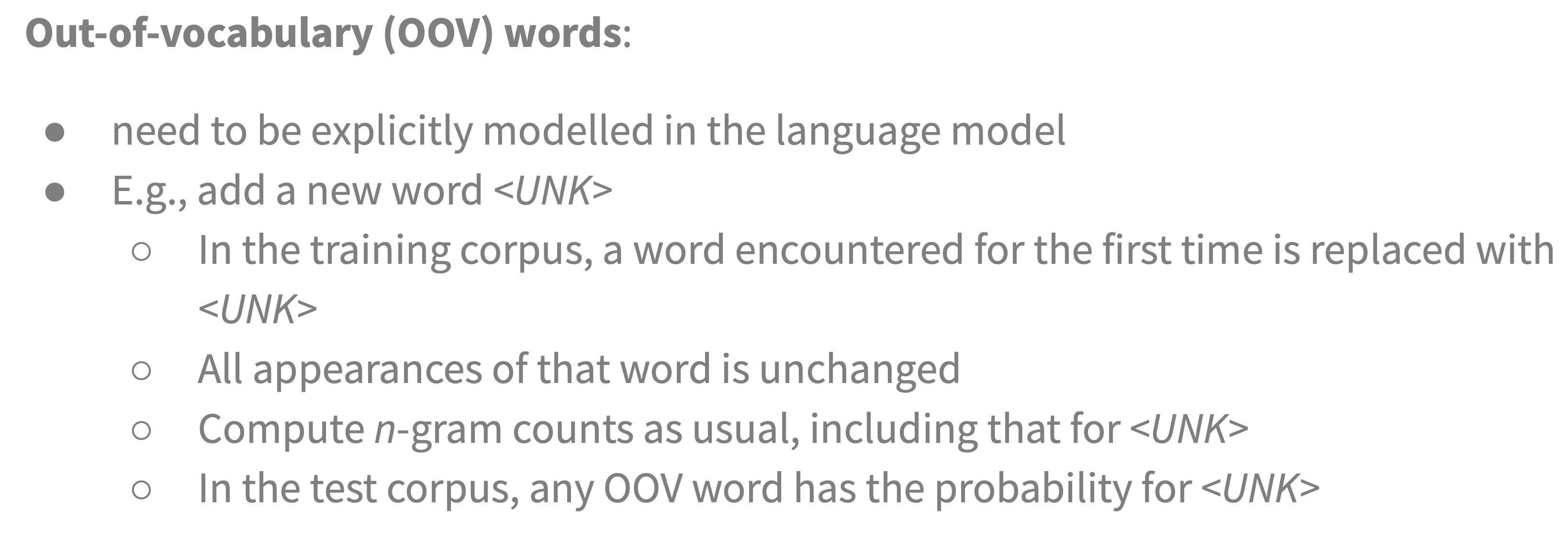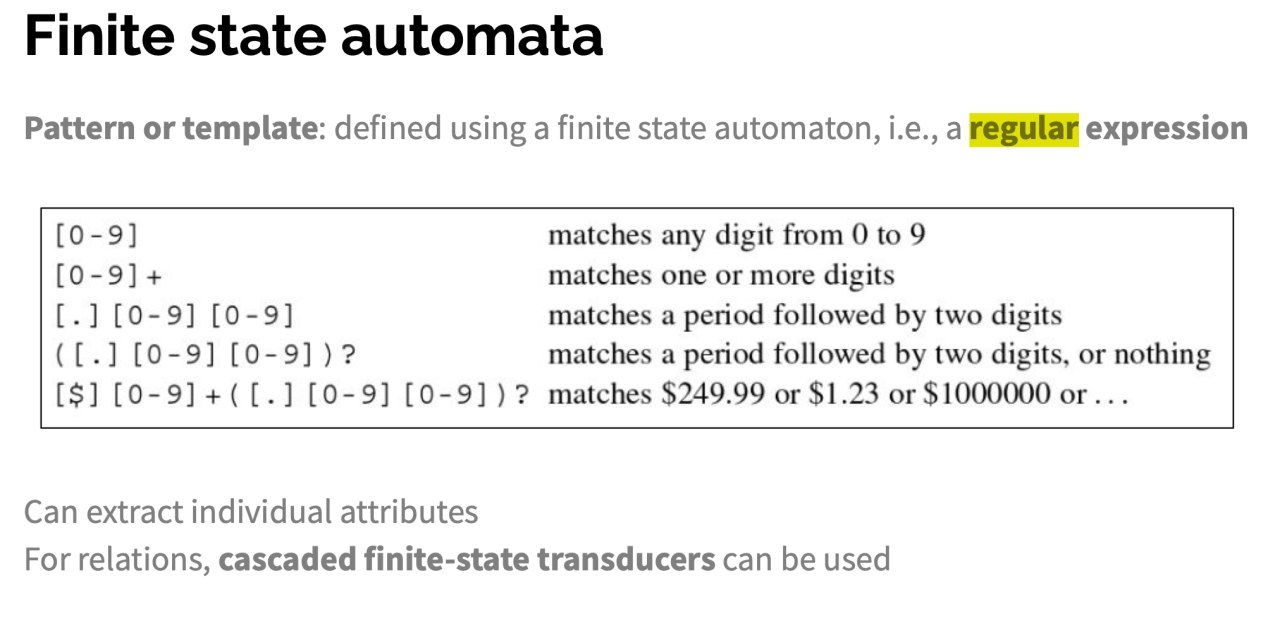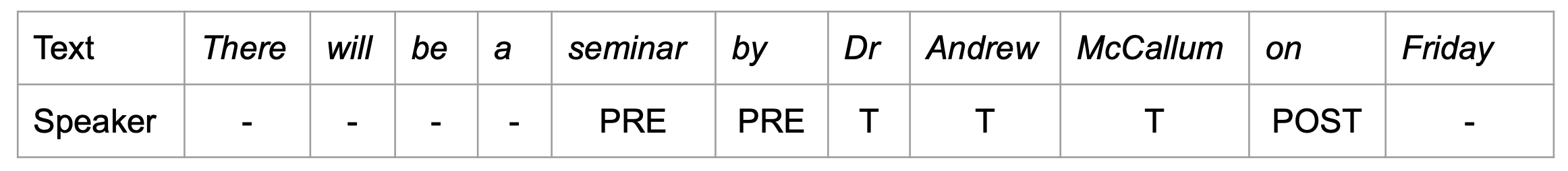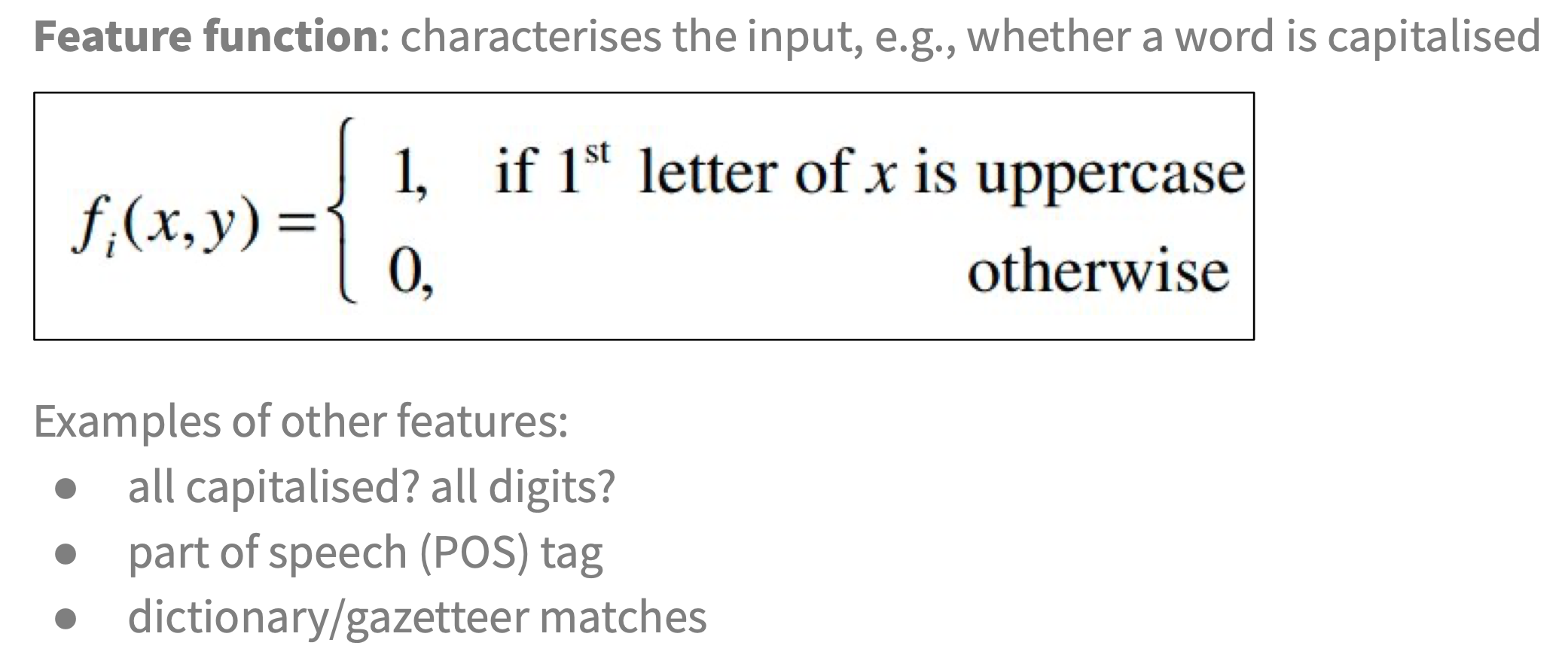# 人工智能导论 自然语言处理

## NLP

Posted by R1NG on December 30, 2021 Viewed Times

# 自然语言处理

## 2. $n$ 元字符模型

$P(c_{1 : N}).$

$n$ 个字符序列上的概率分布 为 $n$ 元模型.其中, 构成自负序列的元素不仅可以是字符, 也可以是 单词, 音节 或其他单元.

$n$ 元模型 (不只局限于 $n$ 元字符模型) 可以定义为 $n-1$ 阶 Markov 链. 显然, 在一个 三元模型 (也就是 $2$ 阶 Markov 链) 中, 我们有:

$P(c_i \vert c_{1 : i-1}) = P(c_i \vert c_{i-2: i-1}).$

$P(c_{1:N}) = \prod_{i=1}^{N}P(c_i \vert c_{1:i-1}) = \prod_{i=1}^{N}P(c_i \vert c_{i-2:i-1}).$

## 3. $n$ 元模型的平滑

$n$ 元模型的问题在于 训练语料只提供了真实概率分布的估计值, 而无法提供所有的边界情况. 因此, 我们需要采取措施使我们的语言模型能够有效地 扩展到在语料库中从未见到过的文本上.

1. Laplace 平滑:

Laplace 平滑指定, 若某个随机布尔变量 $X$ 在目前已有的 $n$ 个观察值中恒为 false, 则

$P(X = \text{true}) = \frac{1}{n+2}.$

换言之, Laplace 平滑假定 多进行两次试验, 可能 一个值为 true, 另一个为 false.

2. 回退模型和线性插值平滑

回退模型的基本思想是: 首先进行 $n$ 元计数统计, 若某些序列的统计值 很低或者为零, 则回退到 $n-1$ 元.

线性插值平滑是一种通过线性插值将 三元, 二元和一元模型 组合起来的后退模型. 线性平滑插值定义概率估计值如下:

$\hat{P}(c_i ~\vert~ c_{i-2 : i-1}) = \lambda_{3} \cdot P(c_i ~\vert~ c_{i-2 : i-1}) + \lambda_{2} \cdot P(c_i ~\vert~ c_{i-1}) + \lambda_{1} \cdot P(c_i).$

此处系数满足

$\lambda_{3} + \lambda_{2} + \lambda_{1} = 1.$

参数值可以是固定的, 也可以通过某些算法进行计算. 关于参数值计算的讨论超出本文讨论的范围, 在此按下不表.

在 $i=1$ 时, 表达式

$P(c_i ~\vert~ c_{i-2 : i-1})$

就退化为

$P(c_1 ~\vert~ c_{-1 : 0}),$

而在 $c_1$ 前并没有字符. 因此, 我们需要引入 人工字符 解决这一问题:

我们可以定义 $c_0$ 为空字符, 也可以回退到 低价 Markov 模型, 定义 $c_{-1 : 0}$ 为 空序列, 从而

$P(c_1 ~\vert~ c_{-1:0}) = P(c_1).$

$n$ 元字符模型可以被进一步扩展为 $n$ 元单词模型, 它检验的最小元素从 字符 变为了 单词. $n$ 元单词模型的平滑方法如下:## 4. 文本分类 (Text Classification)

$P(\text{Message} ~\vert~ \text{spam})$

$P(\text{Message} ~\vert~ \text{ham})$

$\text{argmax}_{c \in \{\text{spam , ham}\}} P(c ~\vert~ \text{Message}) = \text{argmax}_{c \in \{\text{spam , ham}\}} P(\text{Message} ~\vert~ c) \cdot P(c).$

## 5. 信息检索 (Information Retrieval)

1. 文档集合:

每个 IRS 都应确定它需要处理的文档的类型和范围, 如 “一页文本”, “一个段落文本” 还是 “多页文本”.

2. 一个使用某种 查询语言 构造和描述的 查询请求:

查询请求 明确地描述了用户的需求. 组成它的查询语言可以由单词列表, 由多个单词组成的句子, 布尔运算符和非布尔运算符组成.

3. 结果集合:

IRS 应该能够基于特定的查询请求给出对应的查询结果, 它是 文档集合的子集, 包含了与查询请求相关的文档.

4. 结果集合的展示:

IRS 同时应具备向用户展示其检索结果的能力.

### 检索模型

BM25 中, 得分是 由构成查询的每个单词的得分 进行 线性加权 组合而成. 查询项的权重受三个因素的影响:

1. 查询项在文档中出现的频率 TF:

TF 表示 词项频率, 如对于查询 [Long live Axton], 频繁提到 Axton 的文档得分较高.

注意 词项频率 (Term Frequency, TF) 的定义是: 给定的某个单词给定的某篇文章出现的频率:

$\text{TF}(t, d) = \frac{\text{raw count of term t' in document d'}}{\text{document d's word count}}.$

但是在你校的课程中, 词项频率应该被定义为给定的某个单词在某篇文章中出现的次数!

2. 词项的文档频率的倒数 IDF:

如假设单词 long 几乎出现在每个文档中, 则这个词项的 文档频率较高, 进而知其 IDF 较低, 因此 long 没有查询中的 axton 重要.

注意 文档频率 (Document Frequency, DF) 的定义是: 在 全体文章包含给定单词的文档篇数. 它和 TF 是截然不同的两个东西:

$\text{DF}(t, D) = \frac{\text{total number of documents D' which contains term t'}}{\text{total number of documents in corpus D'}}.$

但是在你校的课程中, 文档频率应该被定义为给定的某个单词在全体文章中出现的次数!

同时注意 IDF 并不单纯是 DF 的倒数:

$\text{IDF} = \log_{10}\frac{N - DF + 0.5}{\text{DF + 0.5}}$

注意 IDF 中的平滑 ($+0.5$) 和为了控制数值范围而进行的取对数操作.

3. 文档的长度:

文档的长度越长, 其包含单词的数量越大, 越可能提到所有查询中的单词, 但同时也越可能 携带更多与查询项无关的内容. 因此, 这类文档不一定真正与查询相关. 如果两篇文章都提到了查询中所有的查询项 (查询单词), 则更短的那篇应该作为更佳的相关文档候选.

1. 语料库中的 $N$ 个文档都已经 索引完毕 , 因此对某个单词 $q_i$, 它在文档 $d_j$ 中的 词项频率 $\text{TF}(q_i, d_j)$ 可以被直接从索引中被查找到.

2. 已经构建好了一个 文档频率统计表, 因此对某个单词 $q_i$, 我们可以通过查表得到它在 整个语料库 中的 文档频率 $\text{DF}(q_i)$.

$\text{BM25} (d_j, q_{1:n}) = \sum_{i=1}^{n} \text{IDF}(q_i) \cdot \frac{\text{TF}(q_i, d_j) \cdot (k+1)}{\text{TF}(q_i, d_j) + k \cdot (1-b + b \cdot \frac{\vert d_j \vert}{L})}.$

1. $\vert d_j \vert$ 表示 文档 $d_j$单词计数 的长度 (包含了多少个单词).

2. $L$ 为 语料库中文档平均长度:

$L= \frac{\sum_{k=1}^{N} \vert d_k \vert}{N}.$
1. $k$ 和 $b$ 均为需要结合具体语料库通过交叉验证进行调整的参数. 若未特殊指定, 认为 $k=2, b=0.75$!

### 对检索模型的评价

1. 在结果集合中 的, 和查询 相关 的文档数.
2. 在结果集合中 的, 和查询 不相关 的文档数.
3. 不在结果集合中 的, 和查询 相关 的文档数.
4. 不在结果集合中 的, 和查询 不相关 的文档数.

$F_1$ 值 是 综合准确率和召回率 这两个指标的度量: 它是 准确率和召回率两者几何平均值:

$\text{F}_{1} = \frac{2 \cdot \text{Precision} \cdot \text{RecallRate}}{\text{Precision} + \text{RecallRate}}$

### PageRank 算法

$\text{PR}(p) = \frac{1-d}{N} + d \cdot \sum_{i}\frac{\text{PR}(\text{in}_i)}{C(\text{in}_i)}.$

## 6. 信息提取 (Information Extraction)

### 基于有限状态自动机的信息提取### 信息提取的概率模型

Markov 模型 (HMM) 描述了一个 隐含状态序列, 该序列的每一步都附有一个 观察值 $e_t$. 在这里, 为了将 HMM 应用于信息提取问题上, 我们 为每个属性分别建立一个独立的 HMM.1. HMM概率模型, 因此具有抗噪声的特点, 而在正则表达式中, 只要被检测的句子中哪怕有一个预期的字符丢失了, 正则表达式的匹配也会失败. 而若使用 HMM, 我们就可以很好地对句子中丢失的字符或单词进行 退化处理 (Degradation): 用概率值表示匹配的程度, 而非一刀切地用布尔值表示 “匹配成功” 或 “匹配失败”.

2. HMM 可以通过数据训练得到, 而无需构造复杂的匹配模版. 因此, 这样构造的模型还具有可扩展性的特点, 可以适应 随着时间推移不断变化 的文本.

$P(X_{1:N} ~\vert~ e_{1:N})$

Bayes 网络 类似, 条件随机场也可以表示变量之间的依赖结构. 比如, 线性链条件随机场 (Linear-chain Conditional Random Field) 就可以表示 时间序列中变量之间的 Markov 依赖关系.

$y = \text{argmax}_{y} ~ p_{\lambda}(y ~\vert~ x).$

$p_{\lambda}(y ~\vert~ x) = \frac{1}{Z_x} \cdot \exp(\sum_{i=1}^{n} \sum_{j=1}^{m} \lambda_j \cdot f_j(y_{i-1}, y_i, x, i))$

1. $\frac{1}{Z_x}$ 是确保对所有的 $y$ 而言概率和为 $1$ 的 标准化因子.

2. $\sum_{j=1}^{m} \lambda_j \cdot f_j(y_{i-1}, y_i, x, i)$

特征函数, 显见它被定义为 $m$ 个特征函数 $f_1, \cdots, f_m$ 的加权和. 其中, 参数 $\lambda_j$ 通过最大后验估计过程学习得到.

3. 指数幂上的 $\sum_{i=1}^{n}$ 作用为对标记序列 $y$ 中的每个标记 $y_i$ 求和 (也就是说特征函数要应用在每个标记上).